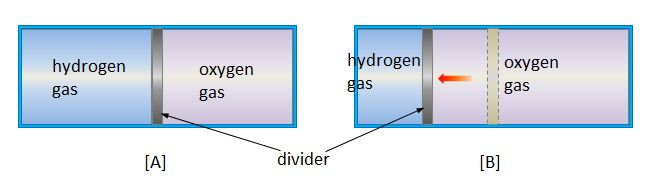# Two gases in containerThe above left is a container with a divider equally dividing hydrogen gas and oxygen gas at $0$°C and $1$ atm. Now, keeping the temperature of both gases at $0$°C, the volume of the hydrogen gas is reduced by half by moving the divider to the left as shown in [B] above. Which of the following statements is correct about the states in [B]? (The molecular weight of an oxygen molecule is $16$ times as large as that of a hydrogen molecule.)

a) The pressure difference between the two gases is $1.5$ atm.

b) The average kinetic energy of the hydrogen molecules is $16$ times as large as that of the oxygen molecules.

c) The average speed of the hydrogen molecules is $4$ times as large as that of the oxygen molecules.

×

Problem Loading...

Note Loading...

Set Loading...